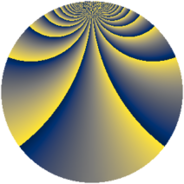# Properties

 Label 2300.2.baLevel $2300$ Weight $2$ Character orbit 2300.ba Rep. character $\chi_{2300}(99,\cdot)$ Character field $\Q(\zeta_{22})$ Dimension $2120$ Sturm bound $720$

# Related objects

## Defining parameters

 Level: $$N$$ $$=$$ $$2300 = 2^{2} \cdot 5^{2} \cdot 23$$ Weight: $$k$$ $$=$$ $$2$$ Character orbit: $$[\chi]$$ $$=$$ 2300.ba (of order $$22$$ and degree $$10$$) Character conductor: $$\operatorname{cond}(\chi)$$ $$=$$ $$460$$ Character field: $$\Q(\zeta_{22})$$ Sturm bound: $$720$$

## Dimensions

The following table gives the dimensions of various subspaces of $$M_{2}(2300, [\chi])$$.

Total New Old
Modular forms 3720 2200 1520
Cusp forms 3480 2120 1360
Eisenstein series 240 80 160

## Trace form

 $$2120q + 10q^{4} - 34q^{6} - 168q^{9} + O(q^{10})$$ $$2120q + 10q^{4} - 34q^{6} - 168q^{9} + 22q^{14} - 26q^{16} - 44q^{21} + 44q^{24} + 10q^{26} + 20q^{29} + 66q^{34} - 46q^{36} - 52q^{41} - 44q^{44} + 22q^{46} + 240q^{49} + 210q^{54} - 22q^{56} - 44q^{61} - 26q^{64} - 88q^{66} + 52q^{69} + 22q^{74} - 132q^{76} - 16q^{81} + 22q^{84} - 242q^{86} + 44q^{89} - 10q^{94} - 300q^{96} + O(q^{100})$$

## Decomposition of $$S_{2}^{\mathrm{new}}(2300, [\chi])$$ into newform subspaces

The newforms in this space have not yet been added to the LMFDB.

## Decomposition of $$S_{2}^{\mathrm{old}}(2300, [\chi])$$ into lower level spaces

$$S_{2}^{\mathrm{old}}(2300, [\chi]) \cong$$ $$S_{2}^{\mathrm{new}}(460, [\chi])$$$$^{\oplus 2}$$